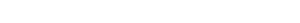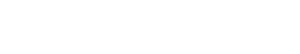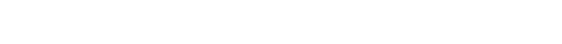# JEE Conic Sections

Conic Sections was once pursued merely to satisfy the craving for knowledge of the ancient Greeks. But it is now an important tool in the study of various branches of engineering. And for aspiring engineering students, it helps you to crack your JEE.

Coordinate geometry carries huge weightage in the JEE mathematics syllabus, and conic sections is an important topic in JEE coordinate geometry. You can expect around two to five questions on this topic in the JEE paper. So, it is very important for you to have good command over this topic if you want to score high. Read on to know some important things about the JEE conic sections!

## Definition

Technically, you would define a conic section as a curve formed by the intersection of a plane with the surface of a double-napped right circular cone. And if you don’t know what a double-napped right circular cone is—let’s make it simple.

Take a right circular cone and stack an inverted right circular cone on its vertex. What you get is a double-napped right circular cone. When you take into account all possible orientations of the intersecting plane with the double-napped cone, you get four curves, namely: a circle, an ellipse, a parabola, and a hyperbola.Image by Wikipedia

There is another definition that you will find more useful for the JEE. A conic section is a locus of all points P such that its distance from a fixed point F (called the focus of the conic), is a constant multiple e, the eccentricity, of the distance from P to a fixed line L, called the directrix of the conic.

This gives us the working mathematical form for a conic section,Now, depending on the value of e, the curves exhibit varying properties. If 0<e<1, we get a closed curve called ellipse. When e=1, we get a parabola and for e >1, we have a hyperbola.

Question 1
Here is a question that will make you more comfortable with the concept.

Given the equationIdentify if it is a
A) Parabola
B) Hyperbola
C) Circle
D) Ellipse

The correct answer is A). It is parabola. With what I told you above, you may find the question mind-boggling! If not, you are really good in algebraic manipulation. Anyway, let me show you how to do it. The aim is to convert the equation 16x2 + 9y2 –92x –256y –24xy + 76 = 0 into generic conic section formula. We proceed as follows.You can compare it with the general form. What you get is focus F ≡(1,2) and the equation of directrix 3x + 4y + 7. Also, the eccentricity is 1. So, you can safely say that it is a parabola.

At times, the necessary factorization may not be trivial. In fact, you may not find one at all. In such a case, we employ a different method. The generic equation for a conic section is a general second-degree equation Ax2 + 2Bxy + Cy2 + 2Dx + 2Ey + F = 0.In the light of the above conditions, you can easily determine what conic section the equation
16x2 + 9y2 –92x –256y –24xy + 76 = 0 represents.
B2 — 4AC = 242 — 4 x 16 x 9 = 576 — 576 = 0. Hence, a parabola.

## Pair of Straight Lines

Now, looking at the general second-order equation, does it remind you of something else you may have studied earlier? To make things more interesting, it is also the equation for a pair of straight lines. You may be wondering how a single equation could represent both the pair of straight lines and conic sections. Well, give the statement some thought.

A pair of straight lines is itself a conic section. Confused? Don’t be. Suppose you have a double-napped right circular cone. Take a plane such that it passes through the vertex of the double-napped cone and also contains its axis. What curve will you get? A pair of straight lines!

For the time being and also for the rest of the article, let us assume that a pair of straight lines and conic sections are two independent entities. Now that you know all this, you want to distinguish a conic section second-order equation from a pair of straight lines second-order equation.

Given an equation ax2 + 2hxy + by2 + 2gx + 2fy + c = 0, it will represent a pair of straight lines if abc + 2fgh — af2 — ch2 — bg2 = 0, otherwise any of the four conic sections.

Question 2
Here is another example for you to practice.

What does the given equation 5x2 — y2 –4xy + 8x — 2y +3 = 0 represent?
A) A pair of straight lines
B) Circle
C) Ellipse
D) Parabola

The correct answer is A) a pair of straight lines. You can use the above formulae to identify the nature of the equation. Also, if you factorize it, you will get (5x + y + 3)(x — y + 1) = 0, which are the equations of the two lines the equation represents.

## Center of Conics

So far, we have only talked about the classification of conic sections. Sometimes, we are also interested in the coordinates of the center of the conic sections. You would ask why? Well, most of the time the properties we are interested in are unaffected by the transformation of coordinates. In such cases, you can shift the center of the conic to a more desirable coordinate to make calculations simple and easy to perform.

Now that you know how you can exploit it, you need to know how to determine the center of a conic. The easiest way to do so is to partially differentiate the conic equation with respect to x and y, and simultaneously solve for x and y from the two equations obtained.

Consider the general equation ax2 + 2hxy + by2 + 2gx + 2fy + c = f(x,y). Therefore,. When you solve for x and y, you get the coordinate of the center of the conic.

## Parameters of Conic Section

When working with conic sections, there are a few terms that you should know.

Principal Axis: In the case of hyperbola and ellipse, there exist two foci—in contrast to the single focus in a parabola. We define the Principal Axis as a straight line perpendicular to the directrix, which passes through the focus (foci).

Focal Parameter: It is the distance between the focus and the directrix.

Focal Length: For a parabola, the focal length is the distance between the focus and the vertex. For ellipse and hyperbola, it is defined as the distance from the center to any of the two foci.

Latus Rectum: The latus rectum of a conic section is the chord (line segment) that passes through the focus, is perpendicular to the major axis, and has both endpoints on the curve. The latus rectum satisfies some properties which are given below:

• The length of the latus rectum of a parabola is four times the focal length.
• In an ellipse, it is two times the square of the length of the minor axis divided by the length of the major axis.
• In a hyperbola, it is two times the square of the length of the transverse axis divided by the length of the conjugate axis.
• For a circle, the latus rectum is its diameter (twice the length of radius).

## Standard Form of Conic Sections

You may find it difficult to wrap your head around the generalized form. Studying their properties will give you a hard time if you are allergic to mathematics. In such cases, the standard form of conics come to the rescue.In all the above equations, the center is (0,0) and the major axis is along x-axis while the minor axis is along y-axis.

In this blog post, we learned about the conic sections, which are present in the JEE maths syllabus. Hope you found it useful. For more amazing JEE study material, check out Magoosh JEE Product. Happy learning!